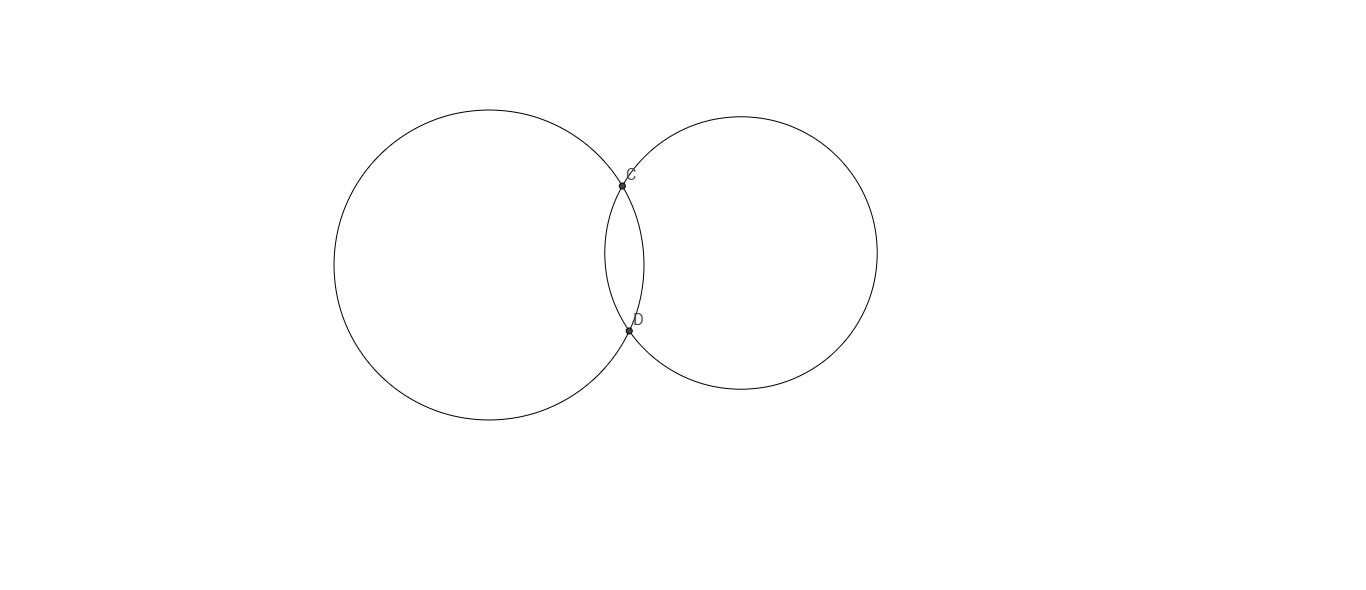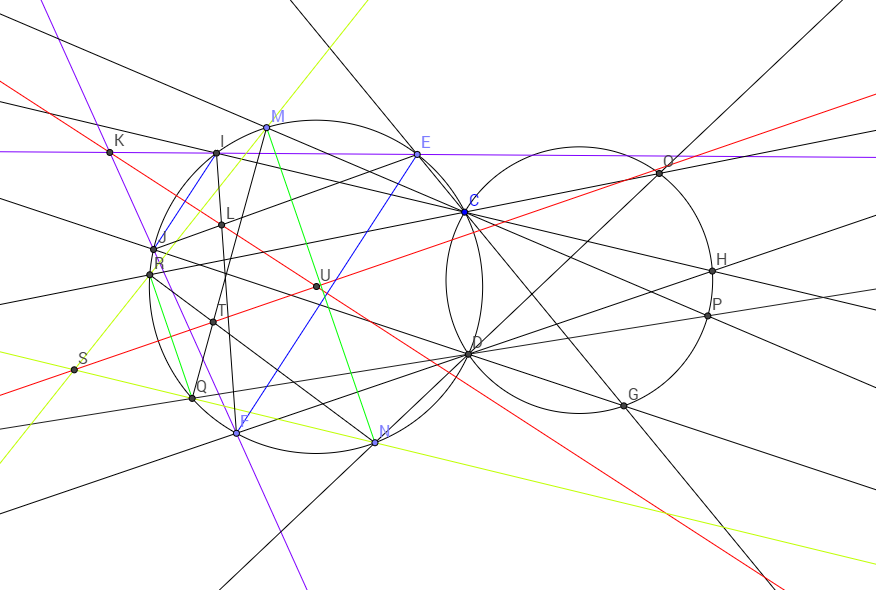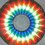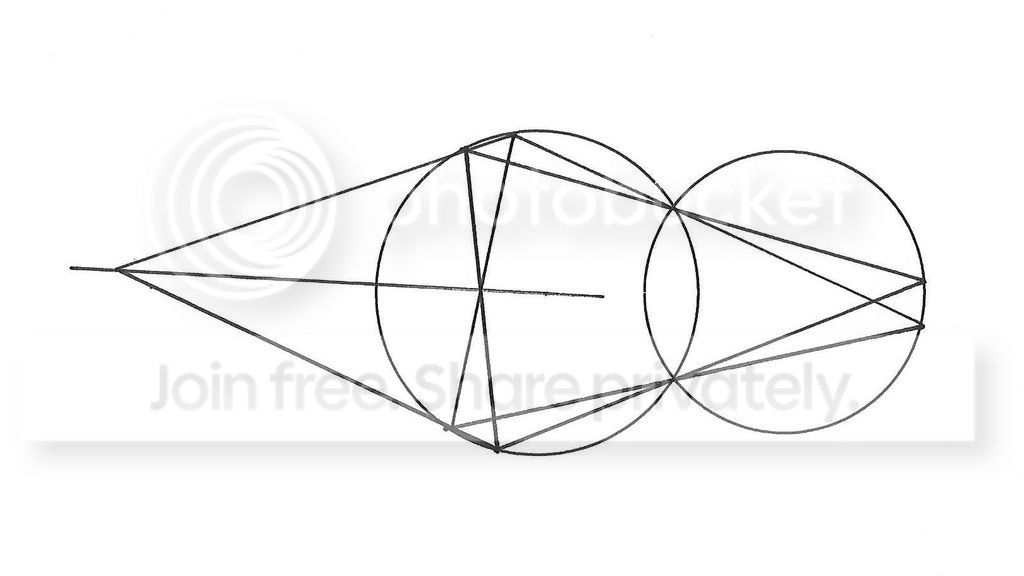# Even More Constructions. This time, it's got some restrictions.I was and still am so glad to get out of school but constructions seem to drag me back there- which is why I try to avoid them. But this one is really interesting and is fun to do:

You are given a sheet of paper with two intersecting circles G1 and G2 with unknown centres. They meet at points C and D. Find their centres using only a straightedge.

A straightedge is a ruler without markings that allows you to join two points by drawing a line through them.

It might help to solve these problems here:

Here is how it was done by me:Here E, F, M and N are the points that I chose. MN and it's parallel line are marked in green and EF and it's parallel line are marked in blue. K and S are the points where the nonparallel sides of the isosceles trapeziums meet. And the lines marked in red are the ones that intersect at the centre U of one of the circles.

I've explained the method in a reply to Michael Mendrin in the comments below.Note by Vishnu C
6 years, 4 months ago

This discussion board is a place to discuss our Daily Challenges and the math and science related to those challenges. Explanations are more than just a solution — they should explain the steps and thinking strategies that you used to obtain the solution. Comments should further the discussion of math and science.

When posting on Brilliant:

• Use the emojis to react to an explanation, whether you're congratulating a job well done , or just really confused .
• Ask specific questions about the challenge or the steps in somebody's explanation. Well-posed questions can add a lot to the discussion, but posting "I don't understand!" doesn't help anyone.
• Try to contribute something new to the discussion, whether it is an extension, generalization or other idea related to the challenge.

MarkdownAppears as
*italics* or _italics_ italics
**bold** or __bold__ bold
- bulleted- list
• bulleted
• list
1. numbered2. list
1. numbered
2. list
Note: you must add a full line of space before and after lists for them to show up correctly
paragraph 1paragraph 2

paragraph 1

paragraph 2

[example link](https://brilliant.org)example link
> This is a quote
This is a quote
    # I indented these lines
# 4 spaces, and now they show
# up as a code block.

print "hello world"
# I indented these lines
# 4 spaces, and now they show
# up as a code block.

print "hello world"
MathAppears as
Remember to wrap math in $$ ... $$ or $ ... $ to ensure proper formatting.
2 \times 3 $2 \times 3$
2^{34} $2^{34}$
a_{i-1} $a_{i-1}$
\frac{2}{3} $\frac{2}{3}$
\sqrt{2} $\sqrt{2}$
\sum_{i=1}^3 $\sum_{i=1}^3$
\sin \theta $\sin \theta$
\boxed{123} $\boxed{123}$

Sort by:

If, by using techniques as described by this problem Can you prove this?, we draw two distinct chords of the same length in one of the circles, it isn't hard to continue using a straightedge only to draw a line through the center of that circle. Repeat using two more distinct chords of the same length, and draw another line through the center. We have marked the center of that circle. Repeat for other circle. That is a lot of straightedge drawing to finally mark both centers but it's indeed possible.

Later maybe I should provide a graphic to show just how messy this can get. I predict it will look like a bird's nest.

What's interesting is that if there was just one circle instead of two intersecting circles, it would be impossible to mark the center of that circle using a straightedge only.

Edit: Here's how it can be done, see sketch done by hand. It was too much work to use graphing software to do this. So, it isn't real precise.Bird's Nest

- 6 years, 4 months ago

How do you get the centre of the circle with two chords of the same length? I don't get it.

Here's how I did it: From Can you prove this?, you have the tools to draw two parallel chords using a straight edge. You extend the nonparallel sides of the isoceles trapezium that you get from there to meet at a point. Next, you do what is done in The Great Divide and you get the mid points of both the chords. Join the midpoints of the chords and you'll find that because the trapezium is isoceles, the line is also perpendicular to the chords.

So now, you repeat the same procedure for another set of parallel chords in the same circle and the meeting points of the perpendicular bisectors of the chords gives you the circle's centre. Do the same for the other circle.

I'm not sure if this is what you did, but I didn't imagine anything nearly as messy as your picture :)

- 6 years, 4 months ago

I just made a figure on geogebra. It's really messy.

- 6 years, 4 months ago

Okay, I'll make up another graphic that shows how it's done for just one circle. If we have 2 crossed chords of equal length, then we can draw 2 more lines passing through the ends of those chords, which will intersect at another point. We then draw a line through both points of intersection, which will pass through the center of the circle. Do this again to get another such line, which will get us the center of the circle where it intersects with the first such line. It's very similar to what you've suggested.

Edit: Here's the graphic, which should be self explanatory.Birds Nest 2

- 6 years, 4 months ago

Is there a way to directly show that the line passes through the centre or is it done by symmetry or the isosceles triangle thing that I explained?

- 6 years, 4 months ago

Symmetry is all you need to demonstrate that the line passing through both points of intersection also passes through the center of the circle. That's why we need 2 chords of EQUAL length.

- 6 years, 4 months ago

I think I got it now. I forgot the exclamation!- 6 years, 4 months ago

Right now, I'm not even sure what disagreement we are even having. Both of our drawings are messy, but the principle is the same.

- 6 years, 4 months ago

If it gets the job done

And I get to have some fun,

Then that's all I care about

And on my face you won't find a pout :)

- 6 years, 4 months ago

What makes this problem particularly interesting for me is that the Poncelet-Steiner Theorem states that given a circle--and its center--then using a straightedge only from there on, one can construct anything that can be done with a compass and straightedge. However, if the center of the circle is not given, it is impossible to find it using a straightedge only. This is one of those classic things in geometry. It was fun to see how this could be done using TWO intersecting circles.

- 6 years, 4 months ago

The two intersecting red lines are the ones that mark the centre U of one of the circles.

- 6 years, 4 months ago